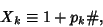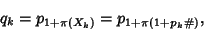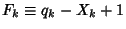## Fortunate PrimeLetwhereis theth Prime andis the Primorial, and letbe the Next Prime (i.e., the smallest Prime greater than),whereis the Prime Counting Function. Then R. F. Fortune conjectured thatis Prime for all. The first values ofare 3, 5, 7, 13, 23, 17, 19, 23, ... (Sloane's A005235), and all known values ofare indeed Prime (Guy 1994). The indices of these primes are 2, 3, 4, 6, 9, 7, 8, 9, 12, 18, .... In numerical order with duplicates removed, the Fortunate primes are 3, 5, 7, 13, 17, 19, 23, 37, 47, 59, 61, 67, 71, 79, 89, ... (Sloane's A046066).

Sloane, N. J. A. Sequences A046066 and A005235/M2418 in An On-Line Version of the Encyclopedia of Integer Sequences.'' http://www.research.att.com/~njas/sequences/eisonline.html and Sloane, N. J. A. and Plouffe, S. The Encyclopedia of Integer Sequences. San Diego: Academic Press, 1995.